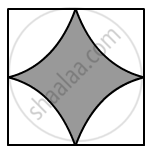Advertisement Remove all ads

# Four Cows Are Tethered at the Four Corners of a Square Field of Side 50 M Such that Each Can Graze the Maximum Unshared Area. What Area Will Be Left Ungrazed? - Mathematics

Sum

Four cows are tethered at the four corners of a square field of side 50 m such that each can graze the maximum unshared area. What area will be left ungrazed?

Advertisement Remove all ads

#### SolutionEach cow can graze a region that cannot be accessed by other cows.

∴ Radius of the region grazed by each cow =50/2 = 25  "m"

Area that each cow grazes=1/4xxpixx"r"^2

= 1/4xx3.14xx25xx25

= 490.625 cm2

Total area grazed = 4 × 490.625 = 1963.49 m

Area of the square = (Side)

= 50

= 2500 cm

Now,

Area left ungrazed= Area of the square - Grazed area

= 2500 - 1963.49 = 536.51 m

Is there an error in this question or solution?
Advertisement Remove all ads

#### APPEARS IN

RS Aggarwal Secondary School Class 10 Maths
Chapter 18 Area of Circle, Sector and Segment
Exercise 18B | Q 25 | Page 832
Advertisement Remove all ads
Advertisement Remove all ads
Share
Notifications

View all notifications

Forgot password?
Course